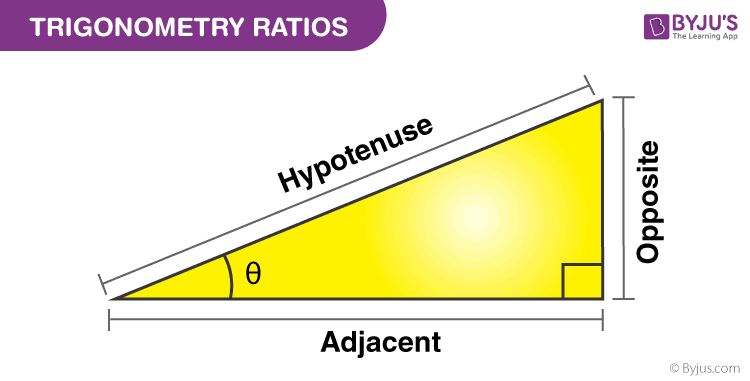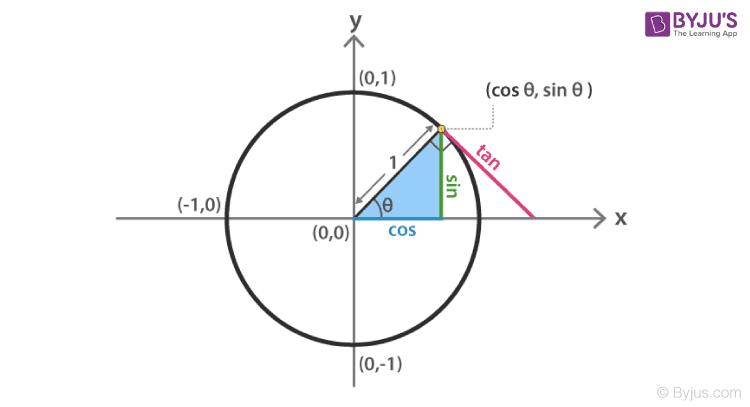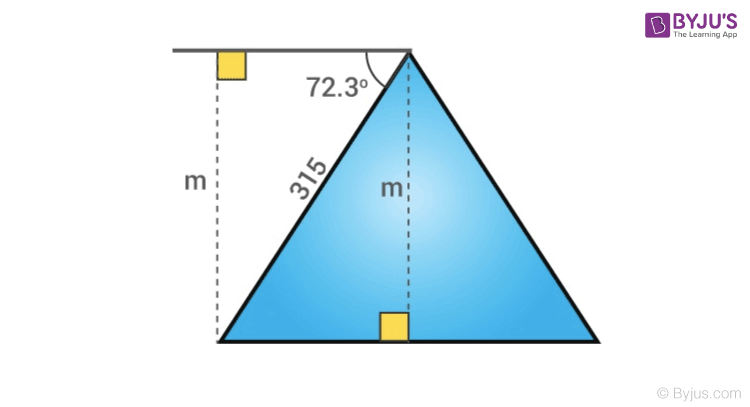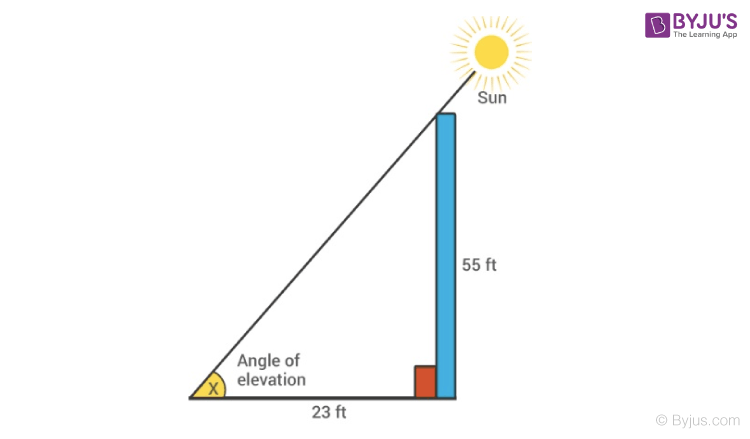# Trigonometry

Trigonometry is one of the important branches in the history of mathematics that deals with the study of the relationship between the sides and angles of a right-angled triangle. This concept is given by the Greek mathematician Hipparchus. In this article, we are going to learn the basics of trigonometry such as trigonometry functions, ratios, trigonometry table, formulas and many solved examples.

## What is Trigonometry?

Trigonometry is one of the most important branches in mathematics that finds huge application in diverse fields. The branch called “Trigonometry” basically deals with the study of the relationship between the sides and angles of the right-angle triangle.  Hence, it helps to find the missing or unknown angles or sides of a right triangle using the trigonometric formulas, functions or trigonometric identities. In trigonometry, the angles can be either measured in degrees or radians. Some of the most commonly used trigonometric angles for calculations are 0°, 30°, 45°, 60° and 90°.

Trigonometry is further classified into two sub-branches. The two different types of trigonometry are:

• Plane Trigonometry
• Spherical Trigonometry

In this article, let us discuss the six important trigonometric functions, ratios, trigonometry table, formulas and identities which helps to find the missing angles or sides of a right triangle.

## Trigonometry Ratios-Sine, Cosine, Tangent

The trigonometric ratios of a triangle are also called the trigonometric functions. Sine, cosine, and tangent are 3 important trigonometric functions and are abbreviated as sin, cos and tan. Let us see how are these ratios or functions, evaluated in case of a right-angled triangle.

Consider a right-angled triangle, where the longest side is called the hypotenuse, and the sides opposite to the hypotenuse are referred to as the adjacent and opposite sides.## Six Important Trigonometric Functions

The six important trigonometric functions (trigonometric ratios) are calculated using the below formulas and considering the above figure. It is necessary to get knowledge about the sides of the right triangle because it defines the set of important trigonometric functions.

 Functions Abbreviation Relationship to sides of a right triangle Sine Function sin Opposite side/ Hypotenuse Tangent Function tan Opposite side / Adjacent side Cosine Function cos Adjacent side / Hypotenuse Cosecant Function cosec Hypotenuse / Opposite side Secant Function sec Hypotenuse / Adjacent side Cotangent Function cot Adjacent side / Opposite side

## Even and Odd Trigonometric Functions

The trigonometric function can be described as being even or odd.

Odd trigonometric functions: A trigonometric function is said to be an odd function if f(-x) = -f(x) and symmetric with respect to the origin.

Even trigonometric functions: A trigonometric function is said to be an even function, if f(-x) = f(x) and symmetric to the y-axis.

We know that

• Sin (-x) = – Sin x
• Cos (-x) = Cos x
• Tan (-x) = -Tan x
• Csc (-x) = – Csc x
• Sec (-x) = Sec x
• Cot (-x) = -Cot x

Therefore, cosine and secant are the even trigonometric functions, whereas sine, tangent, cosecant and cotangent are the odd trigonometric functions. If we know the even and odd trigonometric functions, it helps us to simplify the trigonometric expression when the variable inside the trigonometric function is negative.

## Trigonometry Angles

The trigonometry angles which are commonly used in trigonometry problems are  0°, 30°, 45°, 60° and 90°. The trigonometric ratios such as sine, cosine and tangent of these angles are easy to memorize. We will also show the table where all the ratios and their respective angle’s values are mentioned. To find these angles we have to draw a right-angled triangle, in which one of the acute angles will be the corresponding trigonometry angle. These angles will be defined with respect to the ratio associated with it.

For example, in a right-angled triangle,

Sin θ = Perpendicular/Hypotenuse

or θ = sin-1 (P/H)

Similarly,

θ = cos-1 (Base/Hypotenuse)

θ = tan-1 (Perpendicular/Base)

## Trigonometry Table

Check the table for common angles which are used to solve many trigonometric problems involving trigonometric ratios.

 Angles 0° 30° 45° 60° 90° Sin θ 0 ½ 1/√2 √3/2 1 Cos θ 1 √3/2 1/√2 ½ 0 Tan θ 0 1/√3 1 √3 ∞ Cosec θ ∞ 2 √2 2/√3 1 Sec θ 1 2/√3 √2 2 ∞ Cot θ ∞ √3 1 1/√3 0

In the same way, we can find the trigonometric ratio values for angles beyond 90 degrees, such as 180°, 270° and 360°.

## Unit Circle

The concept of unit circle helps us to measure the angles of cos, sin and tan directly since the centre of the circle is located at the origin and radius is 1. Consider theta be an angle then,Suppose the length of the perpendicular is y and of base is x. The length of the hypotenuse is equal to the radius of the unit circle, which is 1. Therefore, we can write the trigonometry ratios as;

 Sin θ y/1 = y Cos θ x/1 = x Tan θ y/x

## List of Trigonometry Formulas

The Trigonometric formulas or Identities are the equations which are true in the case of Right-Angled Triangles. Some of the special trigonometric identities are given below –

1. Pythagorean Identities
• sin²θ + cos²θ = 1
• tan2θ + 1 = sec2θ
• cot2θ + 1 = cosec2θ
• sin 2θ = 2 sin θ cos θ
• cos 2θ = cos²θ – sin²θ
• tan 2θ = 2 tan θ / (1 – tan²θ)
• cot 2θ = (cot²θ – 1) / 2 cot θ
1. Sum and Difference identities-

For angles u and v, we have the following relationships:

• sin(u + v) = sin(u)cos(v) + cos(u)sin(v)
• cos(u + v) = cos(u)cos(v) – sin(u)sin(v)
• tan(u+v) = $\frac{tan(u)\ +\ tan(v)}{1-tan(u)\ tan(v)}$
• sin(u – v) = sin(u)cos(v) – cos(u)sin(v)
• cos(u – v) = cos(u)cos(v) + sin(u)sin(v)
• tan(u-v) = $\frac{tan(u)\ -\ tan(v)}{1+tan(u)\ tan(v)}$
1. If A, B and C are angles and a, b and c are the sides of a triangle, then,

Sine Laws

• a/sinA = b/sinB = c/sinC

Cosine Laws

• c= a+ b– 2ab cos C
• a= b+ c– 2bc cos A
• b= a+ c– 2ac cos B

### Trigonometry Identities

The three important trigonometric identities are:

• sin²θ + cos²θ = 1
• tan²θ + 1 = sec²θ
• cot²θ + 1 = cosec²θ

### Euler’s Formula for trigonometry

As per the euler’s formula,

eix = cos x + i sin x

Where x is the angle and i is the imaginary number.

$\sin x=\frac{e^{i x}-e^{-i x}}{2 i}\\ \quad \cos x=\frac{e^{i x}+e^{-i x}}{2}\\ \quad \tan x=\frac{\left(e^{i x}-e^{-i x}\right)}{i\left(e^{i x}+e^{-i x}\right)}$

## Trigonometry Basics

The three basic functions in trigonometry are sine, cosine and tangent. Based on these three functions the other three functions that are cotangent, secant and cosecant are derived.

All the trigonometrical concepts are based on these functions. Hence, to understand trigonometry further we need to learn these functions and their respective formulas at first.

If θ is the angle in a right-angled triangle, then

Sin θ = Perpendicular/Hypotenuse

Cos θ = Base/Hypotenuse

Tan θ = Perpendicular/Base

Perpendicular is the side opposite to the angle θ.

The base is the adjacent side to the angle θ.

The hypotenuse is the side opposite to the right angle

The other three functions i.e. cot, sec and cosec depend on tan, cos and sin respectively, such as:

Cot θ = 1/tan θ

Sec θ = 1/cos θ

Cosec θ = 1/sin θ

Hence,

Cot θ = Base/Perpendicualr

Sec θ = Hypotenuse/Base

Cosec θ = Hypotenuse/Perpendicular

## Trigonometry Examples

There are many real-life examples where trigonometry is used broadly.

If we have been given with height of the building and the angle formed when an object is seen from the top of the building, then the distance between object and bottom of the building can be determined by using the tangent function, such as tan of angle is equal to the ratio of the height of the building and the distance. Let us say the angle is ∝, then

Tan ∝ = Height/Distance between object & building

Distance = Height/Tan ∝

Let us assume that height is 20m and the angle formed is 45 degrees, then

Distance = 20/Tan 45°

Since, tan 45° = 1

So, Distance = 20 m

## Applications of Trigonometry

• Its applications are in various fields like oceanography, seismology, meteorology, physical sciences, astronomy, acoustics, navigation, electronics, etc.
• It is also helpful to measure the height of the mountain, find the distance of long rivers, etc.

## Trigonometry Problems and Solutions

Example 1: Two friends, Rakesh and Vishal started climbing a pyramid-shaped hill. Rakesh climbs 315 m and finds that the angle of depression is 72.3 degrees from his starting point. How high is he from the ground?

Solution: Let m is the height above the ground.

To find: Value of mTo solve m, use the sine ratio.

Sin 72.3° = m/315

0.953 = m/315

m= 315 x 0.953

m=300.195 mtr

The man is 300.195 mtr above the ground.

Example 2: A man is observing a pole of height 55 foot. According to his measurement, pole cast a 23 feet long shadow. Can you help him to know the angle of elevation of the sun from the tip of shadow?

Solution:Let x be the angle of elevation of the sun, then

tan x = 55/23 = 2.391

x = tan-1(2.391)

or x = 67.30 degrees

### Trigonometry Questions

Practise these questions given here to get a deep knowledge of Trigonometry. Use the formulas and table given in this article wherever necessary.

Q.1: In △ABC, right-angled at B, AB=22 cm and BC=17 cm. Find:

(a) sin A Cos B

(b) tan A tan B

Q.2: If 12cot θ= 15, then find sec θ.

Q.3: In Δ PQR, right-angled at Q, PR + QR = 30 cm and PQ = 10 cm. Determine the values of sin P, cos and tan P.

Q.4: If sec 4θ = cosec (θ- 300), where 4θ is an acute angle, find the value of A.

## Frequently Asked Questions on Trigonometry

### What do you Mean by Trigonometry?

Trigonometry is one of the branches of mathematics which deals with the relationship between the sides of a triangle (right triangle) with its angles. There are 6 trigonometric functions for which the relation between sides and angles are defined. Learn more about trigonometry now by visiting BYJU’S.

### What are the six basic Trigonometric Functions?

There are 6 trigonometric functions which are:

• Sine function
• Cosine function
• Tan function
• Sec function
• Cot function
• Cosec function

### What is the formula for six trigonometry functions?

The formula for six trigonometry functions are:
Sine A = Opposite side/Hypotenuse
Cos A = Adjacent side / Hypotenuse
Tan A = Opposite side / Adjacent side
Cot A = Adjacent side / Opposite side
Sec A = Hypotenuse / Adjacent side
Cosec A = Hypotenuse / Opposite side

### What is the primary function of trigonometry?

The three primary functions of trigonometry are Sine function, Cosine function and Tangent Function.

### Who is the founder of trigonometry?

A greek astronomer, geographer and mathematician, Hipparchus discovered the concept of trigonometry.

### What are the Applications of Trigonometry in Real Life?

One of the most important real-life applications of trigonometry is in the calculation of height and distance. Some of the sectors where the concepts of trigonometry are extensively used are aviation department, navigation, criminology, marine biology, etc. Learn more about the applications of trigonometry here.

Learn about Trigonometry in a simple manner with detailed information, along with step by step solutions to all questions, only at BYJU’S. Download the app to get personalised videos.

1. Srinivas

It’s a detailed explanation. Helps for my kid exam preparation

2. Gives a very good explanation. Best for the beginner’s as well as professional

3. Nazif gambo

That is my very nice topic in mathematics. Am very happy to this topic tank to all mathemateciat

4. it’s very nice topic in mathematics.

5. Abdussalam auwal kwakwatawa

I very happy with this topic is a very nice topic in mathematics

6. Very nice explain 😃

7. momoh fofanah

So brilliant, well explained.

8. Best way of teaching
Keep it up